Surface Area (Using Formulas) Math Videos for Kids - Grades 6-8
1%
It was processed successfully!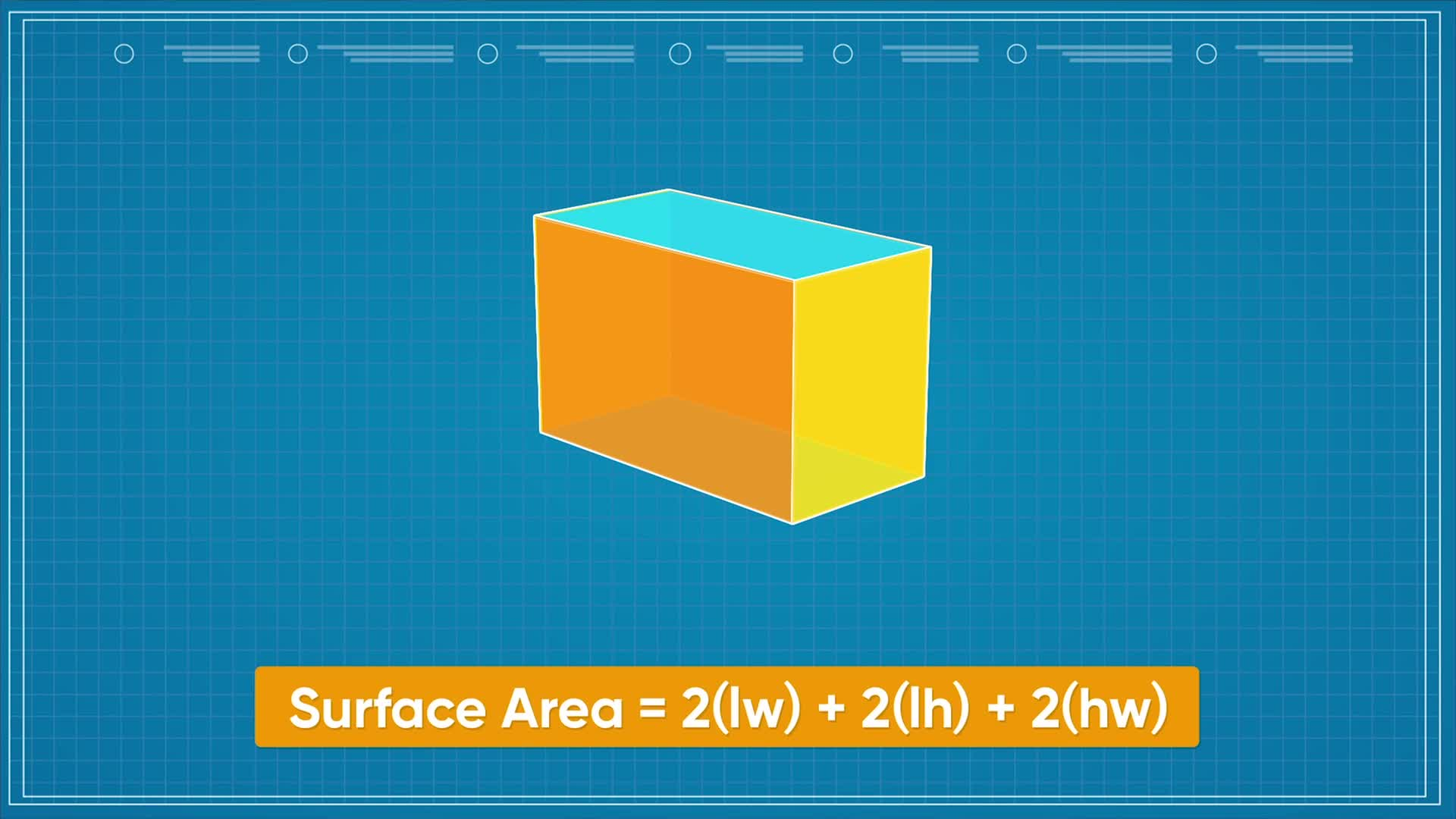WHAT ARE FORMULAS FOR SURFACE AREA?

You have already learned how to find surface area by unfolding 3D shapes into 2D shapes and adding up the areas of all their parts. In this lesson, you learn how to use variables to label the sides in a shape and find surface area more efficiently by using formulas.

To better understand surface area (using formulas)…

WHAT ARE FORMULAS FOR SURFACE AREA?. You have already learned how to find surface area by unfolding 3D shapes into 2D shapes and adding up the areas of all their parts. In this lesson, you learn how to use variables to label the sides in a shape and find surface area more efficiently by using formulas. To better understand surface area (using formulas)…

## LET’S BREAK IT DOWN!

### Surface Area of a Cube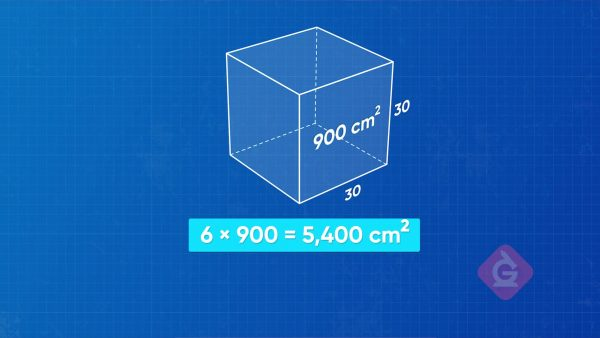A cube is a rectangular prism that has 6 identical faces, and each face is a square. Since all sides have the same length, and all the faces have the same area, we can find the area of one face and multiply by 6 to find the surface area! If we let a be the length of the sides, then we can use the formula 6a2 to find the surface area of any cube. Now you try: What is the area of a cube with side length 4 cm?

Surface Area of a Cube A cube is a rectangular prism that has 6 identical faces, and each face is a square. Since all sides have the same length, and all the faces have the same area, we can find the area of one face and multiply by 6 to find the surface area! If we let a be the length of the sides, then we can use the formula 6a2 to find the surface area of any cube. Now you try: What is the area of a cube with side length 4 cm?

### Surface Area of a Rectangular Prism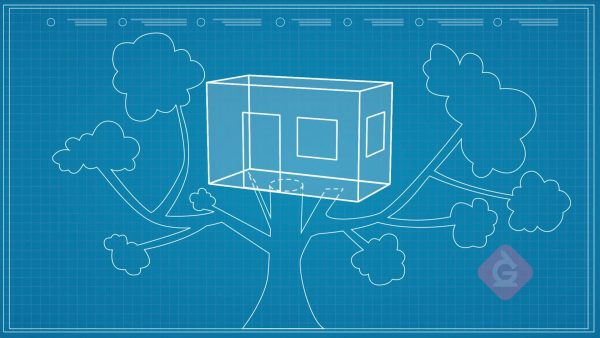A rectangular prism is made up of 6 faces. In a rectangular prism, we have a length, a width, and a height, and we will call them l,w, and h. Since opposite faces have the same dimensions, we only need to find the areas of the 3 different faces, multiply each area by 2, and add the totals together to find the surface area. Then the surface area of a rectangular prism is 2l×w+2w×h+2(l×h). Now you try: Find the surface area of a rectangular prism that has length 6 m, width 3 m, and height 2 m.

Surface Area of a Rectangular Prism A rectangular prism is made up of 6 faces. In a rectangular prism, we have a length, a width, and a height, and we will call them l,w, and h. Since opposite faces have the same dimensions, we only need to find the areas of the 3 different faces, multiply each area by 2, and add the totals together to find the surface area. Then the surface area of a rectangular prism is 2l×w+2w×h+2(l×h). Now you try: Find the surface area of a rectangular prism that has length 6 m, width 3 m, and height 2 m.

### Surface Area of a Triangular Prism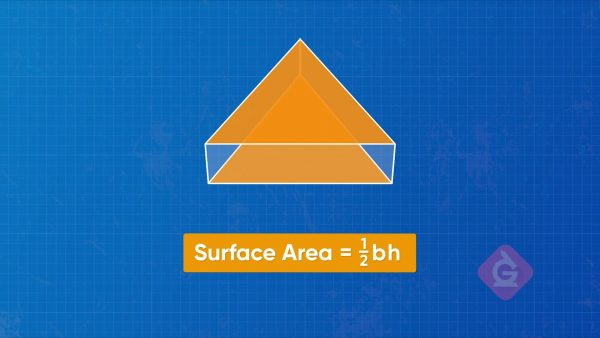A triangular prism has a total of 5 faces: 2 triangular faces that are opposite each other and are the same, and 3 rectangular faces. We can find the area of one of the triangular faces, multiply it by 2, and then add the area of each of the rectangular faces. Then the formula for the surface area of a triangular prism is 2 x [ggfrac]b×h/2[/ggfrac] 2×b × h2+l×a+l×b+l×c which we can also simplify to b×h+l×a+l×b+l×c. It is important to remember that the width might be different for each of the 3 rectangles. Now you try: Find the surface area of a triangular prism where the triangular faces are triangles with side lengths 3 in., 4 in., and 5 in. The triangle base is 3 in. and its height is 4 in., and the rectangular faces have length 2 in.

Surface Area of a Triangular Prism A triangular prism has a total of 5 faces: 2 triangular faces that are opposite each other and are the same, and 3 rectangular faces. We can find the area of one of the triangular faces, multiply it by 2, and then add the area of each of the rectangular faces. Then the formula for the surface area of a triangular prism is 2 x [ggfrac]b×h/2[/ggfrac] 2×b × h2+l×a+l×b+l×c which we can also simplify to b×h+l×a+l×b+l×c. It is important to remember that the width might be different for each of the 3 rectangles. Now you try: Find the surface area of a triangular prism where the triangular faces are triangles with side lengths 3 in., 4 in., and 5 in. The triangle base is 3 in. and its height is 4 in., and the rectangular faces have length 2 in.

### Surface Area of a Cylinder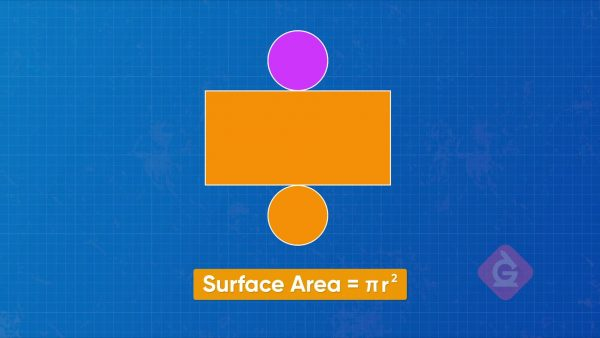A cylinder is like a tube with a circular cap on each end. If we unravel it into a 2D shape, we get one long rectangle and two identical circles. Notice that as we unravel the cylinder, the circumference of the circle turns out to be the length of the rectangle! The other dimension of the rectangle is the height of the cylinder. Then the surface area of a cylinder is 2 times the area of a circle, plus the area of the rectangle. Now you try: Find the surface area of a cylinder that has radius 3 mm and height 6 mm. Use 3.14 for pi and round to the nearest tenth.

Surface Area of a Cylinder A cylinder is like a tube with a circular cap on each end. If we unravel it into a 2D shape, we get one long rectangle and two identical circles. Notice that as we unravel the cylinder, the circumference of the circle turns out to be the length of the rectangle! The other dimension of the rectangle is the height of the cylinder. Then the surface area of a cylinder is 2 times the area of a circle, plus the area of the rectangle. Now you try: Find the surface area of a cylinder that has radius 3 mm and height 6 mm. Use 3.14 for pi and round to the nearest tenth.

### Surface Area of a Cone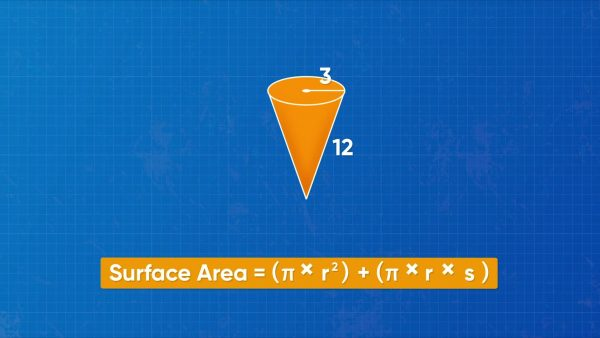Cones are shaped like ice cream cones with a circular lid that covers the opening at the top. An unrolled cone looks like a part of a circle, and the lid is a circle. The unrolled cone can be decomposed into a rectangle that has side lengths πr and s, where s is the slant height of the cone. To find the surface area, we can find the area of the rectangle and add it to the area of the circular lid. Then the formula for surface area of a cone is πr2 + πrs. Now you try: What is the surface area of a cone with radius 4 feet and slant height 7 feet?

Surface Area of a Cone Cones are shaped like ice cream cones with a circular lid that covers the opening at the top. An unrolled cone looks like a part of a circle, and the lid is a circle. The unrolled cone can be decomposed into a rectangle that has side lengths πr and s, where s is the slant height of the cone. To find the surface area, we can find the area of the rectangle and add it to the area of the circular lid. Then the formula for surface area of a cone is πr2 + πrs. Now you try: What is the surface area of a cone with radius 4 feet and slant height 7 feet?

## SURFACE AREA (USING FORMULAS) VOCABULARY

Rectangular Prism
A shape made of 6 rectangular faces. The opposite faces always have the same dimensions.
Cube
A rectangular prism whose sides all have the same length so that all 6 faces are squares.
Face
Each flat part of a shape that we can calculate an area for.
Triangular Prism
A shape that has 2 parallel triangular faces connected by 3 rectangular faces.
Cylinder
A shape that looks like a tube with a circular lid on each end.
Cone
A shape that has a circular base and a slanted edge that extends from around the base to meet at a point.
In a 3D cone or pyramid, the slant height is the length from the edge of base to the point at the top. It is not the same as the actual height of the object.

## SURFACE AREA (USING FORMULAS) DISCUSSION QUESTIONS

### The formula for the surface area of a cube is 6s2. If a side of the cube measures 3 m, how can you find the surface area of the whole cube?

First calculate the power before multiplying by 6. 6s2 means 6 x s x s, and since s = 3, the expression becomes 6(3)2 or 6 x 3 x 3 = 48 m2.

### Can you use the formula for the surface area of a rectangular prism to find the surface area of a cube?

Yes! A cube is a type of rectangular prism. I can use the formula 2l×w+2w×h+2(l×h), but l, w, and h all have the same value, so the result is the same as using for formula 6s2.

### To calculate the surface area of a cylinder, do you need to use πr2 or πr?

I need both! The formula for area of a circle gives the area of the circles at either end of the cylinder. The formula for the circumference of a circle gives the length of the rectangle formed by unrolling the cylinder.

### How should you label a right triangular pyramid so that you can use the surface area formula b×h+a×d+b×d+c×d?

The base of the triangle, b, is also the width of one of the rectangles. In this triangular prism, the height of the triangle, h, is the width of another rectangle, so it could also be labeled a. The third side of the triangle is labeled c, the width of the third rectangle. The dimension d is the height of the prism, and the length of all 3 rectangles.

### The formula for the surface area of a cone uses slant height. What is that?

Slant height is the measurement from the tip of the cone, along the side, down to where the side connects with the circular base. It is different from the actual height of the cone, which is measured at a right angle from the tip to the center of the base.
X

## Success

We’ve sent you an email with instructions how to reset your password.
Ok

## Choose Your Free Trial Period### 7 DaysContinue to Lessons### 90 DaysGet 90 days free by inviting other teachers to try it too.

Share with Teachers

## Get 90 Days Free

### By inviting 4 other teachers to try it too.

4 required

Skip, I will use a 7 days free trial

## Thank You!

Enjoy your free 90 days trial